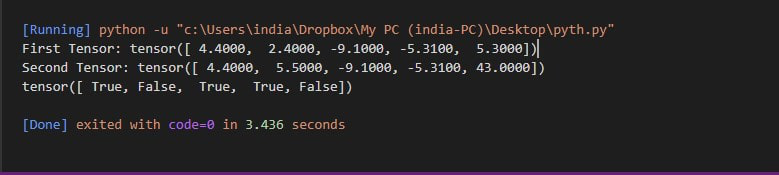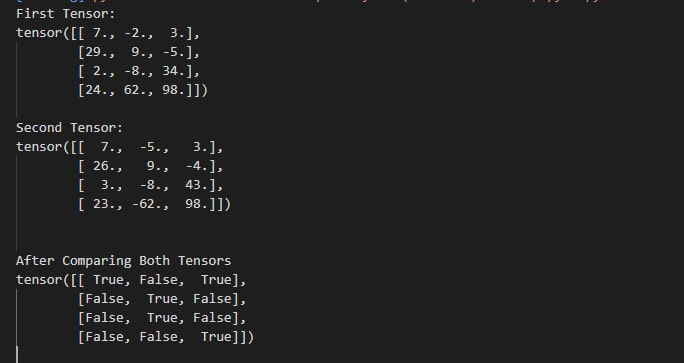Open in App
Not now

# How to compare two tensors in PyTorch?

• Last Updated : 21 Feb, 2022

In this article, we are going to see how we can compare two tensors in Pytorch.

We can compare two tensors by using the torch.eq() method. This method compares the corresponding elements of tensors. It has to return rue at each location where both tensors have equal value else it will return false.

torch.eq() function:

Syntax: torch.eq( First_tensor, Second_tensor, out=None )

Parameters: torch.eq() accept tensors that are we want to compare as parameters.

Return: It return a boolean value. true if tensors are equals else it will return false.

Example 1:

In this example, we are comparing two 1-D tensors using the torch.eq() function in the python programming language .

## Python3

 `# import library ` `import` `torch ` ` `  `# Create first tensor ` `first ``=` `torch.Tensor([``4.4``, ``2.4``, ``-``9.1``,  ` `                      ``-``5.31``, ``5.3``]) ` ` `  `# Create second tensor ` `second ``=` `torch.Tensor([``4.4``, ``5.5``, ``-``9.1``, ` `                       ``-``5.31``, ``43``]) ` ` `  `# print first tensors ` `print``(``"First Tensor:"``, first) ` ` `  `# print first tensors ` `print``(``"Second Tensor:"``, second) ` ` `  `# Compare element wise tensors ` `# first and second ` `print``(torch.eq(first, second)) `

Output:Example 2:

Under this example, we are comparing 2D tensors PyTorch using the torch.eq() function.

## Python3

 `# import library ` `import` `torch ` ` `  `# create two 2D tensors ` `first ``=` `torch.Tensor([[``7``, ``-``2``, ``3``], ` `                      ``[``29``, ``9``, ``-``5``], ` `                      ``[``2``, ``-``8``, ``34``], ` `                      ``[``24``, ``62``, ``98``]]) ` ` `  `second ``=` `torch.Tensor([[``7``, ``-``5``, ``3``], ` `                       ``[``26``, ``9``, ``-``4``], ` `                       ``[``3``, ``-``8``, ``43``], ` `                       ``[``23``, ``-``62``, ``98``]]) ` ` `  `# print first tensors ` `print``(``"First Tensor:"``, first) ` ` `  `# print second tensors ` `print``(``"Second Tensor:\n"``, second) ` ` `  ` `  `print``(``"After Comparing Both Tensors"``) ` ` `  `# Compare element wise tensors first ` `# and second ` `print``(torch.eq(first, second)) `

Output:My Personal Notes arrow_drop_up
Related Articles